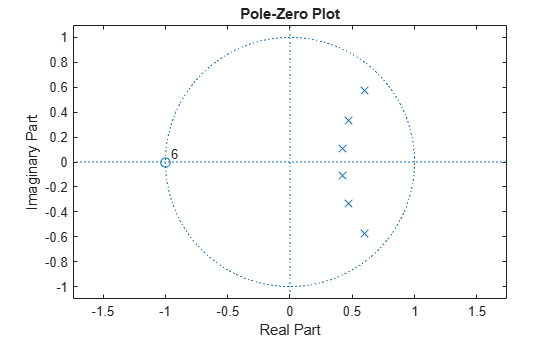# isminphase

Determine whether filter is minimum phase

## Syntax

``flag = isminphase(b,a)``
``flag = isminphase(sos)``
``flag = isminphase(d)``
``flag = isminphase(___,tol)``

## Description

example

````flag = isminphase(b,a)` returns a logical output equal to `1` if the filter specified by numerator coefficients `b` and denominator coefficients `a` is minimum phase.```

example

````flag = isminphase(sos)` returns `1` if the filter specified by second order sections matrix `sos` is minimum phase.```

example

````flag = isminphase(d)` returns `1` if the digital filter `d` is minimum phase. Use `designfilt` to generate `d` based on frequency-response specifications.```

example

````flag = isminphase(___,tol)` uses the tolerance `tol` to determine when two numbers are close enough to be considered equal.```

## Examples

collapse all

Design a sixth-order lowpass Butterworth IIR filter using second order sections. Specify a normalized 3-dB frequency of 0.15. Check if the filter is minimum phase.

```[z,p,k] = butter(6,0.15); SOS = zp2sos(z,p,k); min_flag = isminphase(SOS)```
```min_flag = logical 1 ```

Redesign the filter using `designfilt`. Check that the zeros and poles of the transfer function are on or within the unit circle.

```d = designfilt("lowpassiir",DesignMethod="butter",FilterOrder=6, ... HalfPowerFrequency=0.25); d_flag = isminphase(d)```
```d_flag = logical 1 ```
`zplane(d)`Given a filter defined with a set of single-precision numerator and denominator coefficients, check if it is minimum phase for different tolerance values.

```b = single([1 1.00001]); a = single([1 0.45]); min_flag1 = isminphase(b,a) ```
```min_flag1 = logical 0 ```
`min_flag2 = isminphase(b,a,1e-3)`
```min_flag2 = logical 1 ```

## Input Arguments

collapse all

Filter coefficients, specified as vectors.

Data Types: `single` | `double`

Second order sections, specified as a k-by-6 matrix where the number of sections k must be greater than or equal to 2. Each row of `sos` corresponds to the coefficients of a second order (biquad) filter. The ith row of the `sos` matrix corresponds to `[bi(1) bi(2) bi(3) ai(1) ai(2) ai(3)]`.

Data Types: `double`

Digital filter, specified as a `digitalFilter` object.

Tolerance, specified as a positive scalar. The tolerance value determines when two numbers are close enough to be considered equal.

Data Types: `double`

## Output Arguments

collapse all

Logical output, returned as `1` or `0`. The function returns `1` when the input is a minimum phase filter.

## Version History

Introduced in R2013a